Mathematics: CUET Mock Test - 10

# Mathematics: CUET Mock Test - 10

Test Description

## 40 Questions MCQ Test CUET Science Subjects Mock Tests | Mathematics: CUET Mock Test - 10

Mathematics: CUET Mock Test - 10 for Class 12 2023 is part of CUET Science Subjects Mock Tests preparation. The Mathematics: CUET Mock Test - 10 questions and answers have been prepared according to the Class 12 exam syllabus.The Mathematics: CUET Mock Test - 10 MCQs are made for Class 12 2023 Exam. Find important definitions, questions, notes, meanings, examples, exercises, MCQs and online tests for Mathematics: CUET Mock Test - 10 below.
Solutions of Mathematics: CUET Mock Test - 10 questions in English are available as part of our CUET Science Subjects Mock Tests for Class 12 & Mathematics: CUET Mock Test - 10 solutions in Hindi for CUET Science Subjects Mock Tests course. Download more important topics, notes, lectures and mock test series for Class 12 Exam by signing up for free. Attempt Mathematics: CUET Mock Test - 10 | 40 questions in 45 minutes | Mock test for Class 12 preparation | Free important questions MCQ to study CUET Science Subjects Mock Tests for Class 12 Exam | Download free PDF with solutions
 1 Crore+ students have signed up on EduRev. Have you?
Mathematics: CUET Mock Test - 10 - Question 1

### What will be the average rate of change of the function [y = 16 – x2] between x = 3 and x = 4?

Detailed Solution for Mathematics: CUET Mock Test - 10 - Question 1

Let, y = f(x) = 16 – x2
If x changes from 3 to 4, then, δx = 4 – 3 = 1
Again f(4) = 16 – 42 = 0
And f(3) = 16 – 32 = 7
Therefore, δy = f(4) – f(3) = 0 – 7 = -7
Hence, the average rate of change of the function between x = 3 and x = 4 is:
δy/δx = -7/1 = -7.

Mathematics: CUET Mock Test - 10 - Question 2

### What will be the approximate change in the surface area of a cube of side xm caused by increasing the side by 2%.

Detailed Solution for Mathematics: CUET Mock Test - 10 - Question 2

Let the edge of the cube be x. Given that dx or Δx is equal to 0.02x(2%).
The surface area of the cube is A=6x2
Differentiating w.r.t x, we get
dA/dx =12x
dA= (dA/dx)Δx=12x(0.02x)=0.24x2
Hence, the approximate change in volume is 0.24x2.

Mathematics: CUET Mock Test - 10 - Question 3

### Find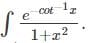Detailed Solution for Mathematics: CUET Mock Test - 10 - Question 3

Let −cot−1x=t
Differentiating w.r.t x, we get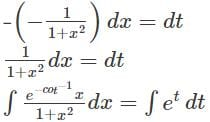=et
Replacing t with -cot-1x, we get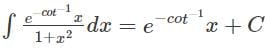Mathematics: CUET Mock Test - 10 - Question 4

Find the integral of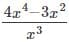.

Detailed Solution for Mathematics: CUET Mock Test - 10 - Question 4

To find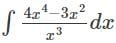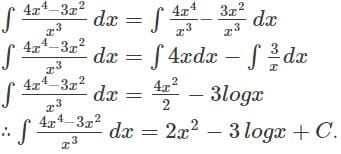Mathematics: CUET Mock Test - 10 - Question 5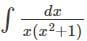equals ______

Detailed Solution for Mathematics: CUET Mock Test - 10 - Question 5

We know that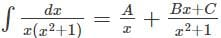By simplifying it we get,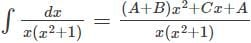Now equating the coefficients we get A = 0, B = 0, C=1.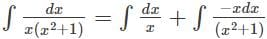Therefore after integrating we get log|x|– (1/2)log(x2+1)  + C.

Mathematics: CUET Mock Test - 10 - Question 6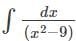equals ___

Detailed Solution for Mathematics: CUET Mock Test - 10 - Question 6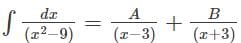By simplifying, it we get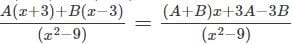By solving the equations, we get, A+B=0 and 3A-3B=1
By solving these 2 equations, we get values of A=1/6 and B=-1/6.
Now by putting values in the equation and integrating it we get value,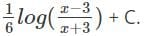Mathematics: CUET Mock Test - 10 - Question 7

What will be the average rate of change of the function [y = 16 – x2] at x = 4?

Detailed Solution for Mathematics: CUET Mock Test - 10 - Question 7

Let, y = f(x) = 16 – x2
dy/dx = -2x
Now, [dy/dx]x = 4 = [-2x]x = 4
So, [dy/dx]x = 4 = -8

Mathematics: CUET Mock Test - 10 - Question 8

Find the approximate value of f(4.04), where f(x)=7x3+6x2-4x+3.

Detailed Solution for Mathematics: CUET Mock Test - 10 - Question 8

Let x=4 and Δx=0.04
Then, f(x+Δx)=7(x+Δx)3+7(x+Δx)2-4(x+Δx)+3
Δy=f(x+Δx)-f(x)
∴f(x+Δx)=Δy+f(x)
Δy=f'(x)Δx
⇒ f(x+Δx)=f(x)+f’ (x)Δx
Here, f'(x)=21x2+12x-4
f(4.04)=(7(4)3+6(4)2-4(4)+3)+(21(4)2+12(4)-4)(0.04)
f(4.04)=(7(4)3+6(4)2-4(4)+3)+(21(4)2+12(4)-4)(0.04)
f(4.04)=531+380(0.04)=546.2

Mathematics: CUET Mock Test - 10 - Question 9

Find the integral of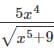.

Detailed Solution for Mathematics: CUET Mock Test - 10 - Question 9

Let x5+9=t
Differentiating w.r.t x, we get
5x4 dx=dt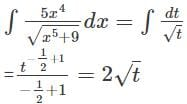Replacing t with x5+9, we get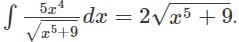Mathematics: CUET Mock Test - 10 - Question 10

Find ∫3cosx+(1/x)dx .

Detailed Solution for Mathematics: CUET Mock Test - 10 - Question 10

To find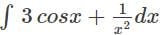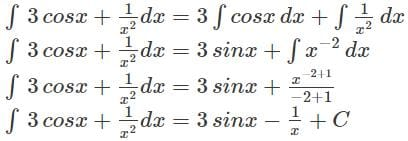Mathematics: CUET Mock Test - 10 - Question 11

What will be the value of the co-ordinate whose position of a particle moving along the parabola y2 = 4x at which the rate at of increase of the abscissa is twice the rate of increase of the ordinate?

Detailed Solution for Mathematics: CUET Mock Test - 10 - Question 11

Here, y2 = 4x ….(1)
Let, (x, y) be the position of the particle moving along the parabola (1) at time t.
Now, differentiating both sides of (1) with respect to t, we get:
2y(dy/dt) = 4(dx/dt)
Or, y(dy/dt) = 2(dy/dt) ……….(2)
By question, dx/dt = 2 * dy/dt ……….(3)
From (2) and (3) we get, y(dy/dt) = 2 * 2 dy/dt
Or, y = 4
Putting y = 4 in (1) we get, 42 = 4x
So, x = 4
Thus, the co-ordinate of the particle is (4, 4).

Mathematics: CUET Mock Test - 10 - Question 12

Find the approximate value of (127)1/3.

Detailed Solution for Mathematics: CUET Mock Test - 10 - Question 12

Let y=(x)1/3. Let x=125 and Δx=2
Then, Δy=(x+Δx)1/3-x1/3
Δy=(127)1/3-(125)1/3
(127)1/3=Δy+5
dy is approximately equal to Δy is equal to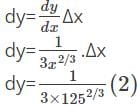dy=2/75=0.0267
∴ The approximate value of (127)1/3 is 5+0.0267=5.0267

Mathematics: CUET Mock Test - 10 - Question 13

Find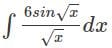Detailed Solution for Mathematics: CUET Mock Test - 10 - Question 13

Let √x = t
Differentiating w.r.t x,we get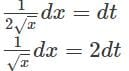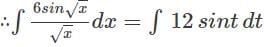=12(-cos⁡t)=-12 cos⁡t
Replacing t with √x, we get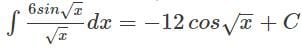Mathematics: CUET Mock Test - 10 - Question 14

Find ∫(2+x)x √x dx .

Detailed Solution for Mathematics: CUET Mock Test - 10 - Question 14

To find ∫(2+x) x √x dx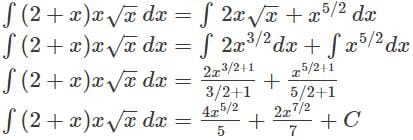Mathematics: CUET Mock Test - 10 - Question 15

Which form of rational function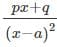represents?

Detailed Solution for Mathematics: CUET Mock Test - 10 - Question 15

It is a form of the given partial fraction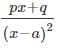which can also be written as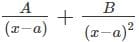and is further used to solve integration by partial fractions numerical.

Mathematics: CUET Mock Test - 10 - Question 16

The time rate of change of the radius of a sphere is 1/2π. When it’s radius is 5cm, what will be the rate of change of the surface of the sphere with time?

Detailed Solution for Mathematics: CUET Mock Test - 10 - Question 16

Let, r be the radius and s be the area of the surface of the sphere at time t.
By question, dr/dt = 1/2π
Now, s = 4πr2;
Thus, ds/dt = 4π * 2r(dr/dt) = 8πr(dr/dt)
When r = 5cm and dr/dt = 1/2π
Then ds/dt = 8π*5*(1/2π) = 20
Thus, correct answer is 20 sq cm.

Mathematics: CUET Mock Test - 10 - Question 17

Find the approximate change in the volume of cube of side xm caused by increasing the side by 6%.

Detailed Solution for Mathematics: CUET Mock Test - 10 - Question 17

We know that the volume V of a cube is given by V=x3
Differentiating w.r.t x, we get
dV/dx = 3x2
dV = (dV/dx)Δx=3x2 Δx
dV=3x2 (6x/100)=0.18x3
Therefore, the approximate change in volume is 0.18x3.

Mathematics: CUET Mock Test - 10 - Question 18

Find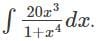Detailed Solution for Mathematics: CUET Mock Test - 10 - Question 18

Let 1+x4=t
4x3 dx=dt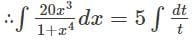=5 log⁡t
Replacing t with 1+x4, we get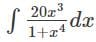= 5log(1+x4)+C

Mathematics: CUET Mock Test - 10 - Question 19

Find ∫7x8−4e2x− (2/x2)dx .

Detailed Solution for Mathematics: CUET Mock Test - 10 - Question 19

To find: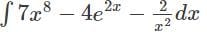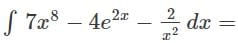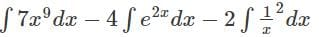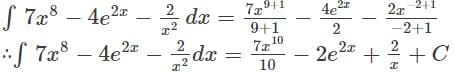Mathematics: CUET Mock Test - 10 - Question 20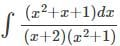equals ______

Detailed Solution for Mathematics: CUET Mock Test - 10 - Question 20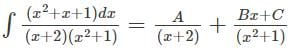Now equating, (x2+x+1) = A (x2+1) + (Bx+C) (x+2)
After equating and solving for coefficient we get values,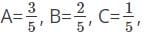now putting these values in the equation we get,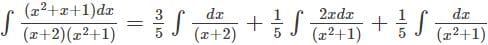Hence it comes,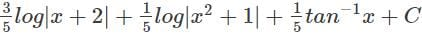Mathematics: CUET Mock Test - 10 - Question 21

A solid cube changes its volume such that its shape remains unchanged. For such a cube of unit volume, what will be the value of rate of change of volume?

Detailed Solution for Mathematics: CUET Mock Test - 10 - Question 21

Let x be the length of a side of the cube.
If v be the volume and s the area of any face of the cube, then
v = x3 and s = x2
Thus, dv/dt = dx3/dt = 3x2 (dx/dt)
And ds/dt = dx2/dt = 2x(dx/dt)
Now, (dv/dt)/(ds/dt) = 3x/2
Or, dv/dt = (3x/2)(ds/dt)
Now, for a cube of unit volume we have,
v = 1
⇒ x = 1 [as, x is real]
Therefore, for a cube of unit volume [i.e. for x = 1], we get,
dv/dt = (3/2)(ds/dt)
Thus the rate of change of volume = 3/2*(rate of change of area of any face of the cube)

Mathematics: CUET Mock Test - 10 - Question 22

Find the approximate value of (82)1/4.

Detailed Solution for Mathematics: CUET Mock Test - 10 - Question 22

Let y=x1/4. Let x=81 and Δx=1
Then, Δy=(x+Δx)1/4-x1/4
Δy=821/4-811/4
821/4=Δy+3
dy is approximately equal to Δy is equal to
dy = (dy/dx)Δx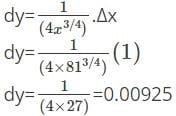∴ The approximate value of 821/4 is 3+0.00925=3.00925

Mathematics: CUET Mock Test - 10 - Question 23

Integrate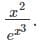Detailed Solution for Mathematics: CUET Mock Test - 10 - Question 23

Let x3=t
3x2 dx=dt
x2 dx=dt/3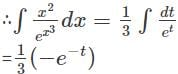Replacing t with x3, we get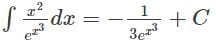Mathematics: CUET Mock Test - 10 - Question 24

Find the integral ∫sin2x+e3x−cos3xdx.

Detailed Solution for Mathematics: CUET Mock Test - 10 - Question 24

To find ∫sin2x+e3x−cos3xdx
∫sin2x+e3x−cos3xdx=∫sin2xdx+∫e3xdx−∫cos3xdx
∫sin2x+e3x−cos3xdx=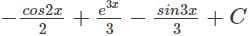Mathematics: CUET Mock Test - 10 - Question 25

Identify the type of the equation (x+1)2.

Detailed Solution for Mathematics: CUET Mock Test - 10 - Question 25

As it represents the identity (b+a)2 it satisfies the identity (b+a)2 = (a2 + b2 +2ab) and is not linear, cubic or an imaginary equation so the correct option is Identity Equation.

Mathematics: CUET Mock Test - 10 - Question 26

A 5 ft long man walks away from the foot of a 12(½) ft high lamp post at the rate of 3 mph. What will be the rate at which the shadow increases?

Detailed Solution for Mathematics: CUET Mock Test - 10 - Question 26

Let, AB be the lamp-post whose foot is A, and B is the source of light, and given (AB)’ = 12(½) ft.
Let MN denote the position of the man at time t where (MN)’ = 5ft.
Join BN and produce it to meet AM(produced) at P.
Then the length of man’s shadow= (MP)’
Assume, (AM)’ = x and (MP)’ = y. Then, (PA)’ = (AM)’ + (MP)’ = x + y
And dx/dt = velocity of the man = 3
Clearly, triangles APB and MPN are similar.
Thus, (PM)’/(MN)’ = (PA)’/(AB)’
Or, y/5 = (x + y)/12(½)
Or, (25/2)y = 5x + 5y
Or, 3y = 2x
Or, y = (2/3)x
Thus, dy/dt = (2/3)(dx/dt)
As, dx/dt = 2,
= 2/3*3 = 2mph

Mathematics: CUET Mock Test - 10 - Question 27

Find the approximate error in the volume of the sphere if the radius of the sphere is measured to be 6cm with an error of 0.07cm.

Detailed Solution for Mathematics: CUET Mock Test - 10 - Question 27

Let x be the radius of the sphere.
Then, x=6cm and Δx=0.07cm
The volume of a sphere is given by V= (4/3)πx3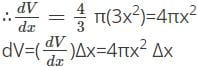dV=4×π×62×0.07
dV=10.08π cm3

Mathematics: CUET Mock Test - 10 - Question 28

Find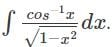Detailed Solution for Mathematics: CUET Mock Test - 10 - Question 28

Let cos-1⁡x=t
Differentiating w.r.t x, we get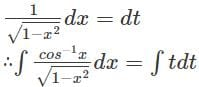= t2/2
Replacing t with cos-1x,we get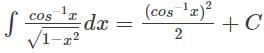Mathematics: CUET Mock Test - 10 - Question 29

Find the integral of (ax2+b)2.

Detailed Solution for Mathematics: CUET Mock Test - 10 - Question 29

To find (ax2+b)2
∫(ax2+b)2dx=∫(a2x4+b2+2ax2b)dx
∫(ax2+b)2dx=∫a2x4dx+∫b2dx+2∫ax2bdx
∫(ax2+b)2dx=a2∫x4dx+b2∫dx+2ab∫x2dx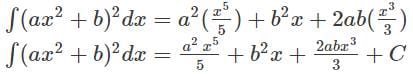Mathematics: CUET Mock Test - 10 - Question 30

For the given equation (x+2) (x+4) = x2 + 6x + 8, how many values of x satisfies this equation?

Detailed Solution for Mathematics: CUET Mock Test - 10 - Question 30

If we solve the L.H.S. (Left Hand Side) of the equation, we get the following value.
(x+2) (x+4) = x2 + 4x + 2x + 8 = x2 + 6x + 8.
This value is same as the R.H.S. (Right Hand Side).
So, all the values of x satisfy the equality.

Mathematics: CUET Mock Test - 10 - Question 31

A ladder 20 ft long leans against a vertical wall. If the top end slides downwards at the rate of 2ft per second, what will be the rate at which the slope of the ladder changes?

Detailed Solution for Mathematics: CUET Mock Test - 10 - Question 31

Let the height on the wall be x and laser touches the ground at distance y from the wall. The length of the ladder is 20ft.
By Pythagoras theorem:
x2 + y2 = 400
Differentiating with respect to t:
2x(dx/dt) + 2y(dy/dt) = 0
Dividing throughout by 2:
x (dx/dt) + y (dy/dt) = 0
Now, dx/dt = -2 ft /s. negative because downwards
x(-2) + y (dy/dt) = 0 ………..(1)
When lower end is 12 ft from wall, let us find x:
x2 + 122 = 400
x2 = 400 – 144= 256
x = 16
x(2) + y (dy/dt) = 0 from (1)
16(-2) + 12 (dy/dt) = 0
-32 + 12(dy/dt) = 0
dy/dt = (32/12) = (8/3)
Thus, lower end moves on a horizontal floor when it is 12 ft from the wall at the rate of 8/3 ft/s
Now, assume that the ladder makes an angle θ with the horizontal plane at time t.
If, m be the slope of the ladder at time t, then,
m = tanθ = x/y
Thus, dm/dt = d/dt(x/y) = [y(dx/dt) – x(dx/dt)]/y2
Therefore, the rate of change of slope of ladder is,
[dm/dt]y = 2 = [12*(-2) – 16*(8/3)]/(12)2
Now, putting the value of x = 16, when y = 12 and dx/dt = -2, dy/dt = 8/3
We get, [dm/dt]y = 12 = [12(-2) – 16(8/3)]/(12)2 = -25/54

Mathematics: CUET Mock Test - 10 - Question 32

A particle moving in a straight line covers a distance of x cm in t second, where x = t3 + 6t2 – 15t + 18. What will be the acceleration of the particle at the end of 2 seconds?

Detailed Solution for Mathematics: CUET Mock Test - 10 - Question 32

We have, x = t3 + 6t2 – 15t + 18
Let, ‘v’ be the velocity of the particle and ‘a’ be the acceleration of the particle at the end of t seconds. Then,
v = dx/dt = d/dt(t3 + 6t2 – 15t + 18)
So, v = 3t2 + 12t – 15
Therefore, a = dv/dt = d/dt(3t2 + 12t – 15)
So, a = 6t + 12
Thus, acceleration of the particle at the end of 2 seconds is, [dv/dt]t = 2 = 6(2) + 12 = 24cm/sec2.

Mathematics: CUET Mock Test - 10 - Question 33

Water is flowing into a right circular conical vessel, 45 cm deep and 27 cm in diameter at the rate of 11 cc per minute. How fast is the water level rising when the water is 30 cm deep?

Detailed Solution for Mathematics: CUET Mock Test - 10 - Question 33

Let ‘r’ be the radius and ‘h’ be the height of the water level at time t.
Then the volume of water level ‘V’ at time t is given by,
V = 1/3 (πr2h) ……….(1)
Given the radius of the base of the cone is = (OA)’ = 27/2 cm and its height = (OB)’ = 45cm.
Again at time t, the radius of the water level = r = (CD)’ and its height = h = (CB)’
Clearly, the triangle OAB and CBD are similar.
Therefore, (CD)’/(CB)’ = (OA)’/(OB)’
Or, r/h = (22/7)/45 = 3/10
or, r = 3h/10
Thus, from (1) we get,
V = 1/3 π (3h/10)2 h = (3π/100)h3
Thus, dV/dt = (3π/100)*3h2(dh/dt)
= (9πh2/100)(dh/dt)
When, h = 30cm, then, 11 = (9π/100) (30)2 (dh/dt) [as for all the values of t we have, dV/dt = 11]
Or, dh/dt = (11/(9π*9)) = 0.043
Thus, the rising rate of rising is 0.043 cm/minute.

Mathematics: CUET Mock Test - 10 - Question 34

A particle moving in a straight line covers a distance of x cm in t second, where x = t3 + 6t2 – 15t + 18. When does the particle stop?

Detailed Solution for Mathematics: CUET Mock Test - 10 - Question 34

We have, x = t3 + 6t2 – 15t + 18
The particle stops when dx/dt = 0
And, dx/dt = d/dt(t3 + 6t2 – 15t + 18)
3t2 + 12t – 15 = 0
⇒ t2 + 4t –5 = 0
⇒ (t – 1)(t + 5)= 0
Thus, t = 1 or t = -5
Hence, the particle stops at the end of 1 second.

Mathematics: CUET Mock Test - 10 - Question 35

A particle moving in a straight line covers a distance of x cm in t second, where x = t3 + 6t2 – 15t + 18. What will be the velocity of the particle at the end of 2 seconds?

Detailed Solution for Mathematics: CUET Mock Test - 10 - Question 35

We have, x = t3 + 6t2 – 15t + 18
Let, v be the velocity of the particle at the end of t seconds. Then, v = dx/dt = d/dt(t3 + 6t2 – 15t + 18)
So, v = 3t2 + 12t – 15
Thus, velocity of the particle at the end of 2 seconds is, [dx/dt]t = 2 = 3(2)2 + 12(2) – 15 = 21cm/sec.

Mathematics: CUET Mock Test - 10 - Question 36

If L1 and L2 have the direction ratios a1,b1,cand a2,b2,c2 respectively then what is the angle between the lines?

Detailed Solution for Mathematics: CUET Mock Test - 10 - Question 36

If L1 and L2 have the direction ratios
a1,b1,cand a2,b2,c2  respectively then the angle between the lines is given by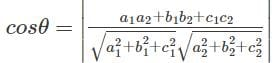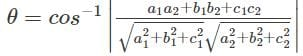Mathematics: CUET Mock Test - 10 - Question 37

Find the value of p such that the lines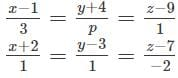are at right angles to each other.

Detailed Solution for Mathematics: CUET Mock Test - 10 - Question 37

The angle between two lines is given by the equation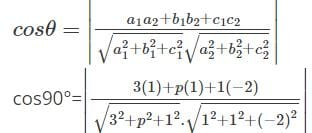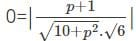0 = p+1
p = -1

Mathematics: CUET Mock Test - 10 - Question 38

Find the value of p such that the lines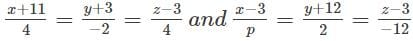are at right angles to each other.

Detailed Solution for Mathematics: CUET Mock Test - 10 - Question 38

We know that, if two lines are perpendicular to each other then, a1a2 + b1b2 + c1c2 = 0
i.e.4(p)+(-2)2+4(-12)=0
4p-4-48=0
4p=52
p = 52/4 = 13.

Mathematics: CUET Mock Test - 10 - Question 39

If the equations of two lines L1 and L2 are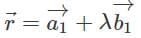and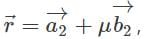then which of the following is the correct formula for the angle between the two lines?

Detailed Solution for Mathematics: CUET Mock Test - 10 - Question 39

Given that the equations of the lines are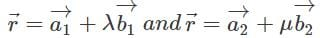∴ the angle between the two lines is given by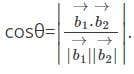Mathematics: CUET Mock Test - 10 - Question 40

If two lines L1 and L2 are having direction cosines l1,m1,n1and l2,m2,n2 respectively, then what is the angle between the two lines?

Detailed Solution for Mathematics: CUET Mock Test - 10 - Question 40

If two lines L1 and L2 are having direction cosines l1,m1,nand l2,m2,n2  respectively, then the angle between the lines is given by cos⁡θ=|l1l2+m1m2+n1n2|

## CUET Science Subjects Mock Tests

60 tests
 Use Code STAYHOME200 and get INR 200 additional OFF Use Coupon Code
Information about Mathematics: CUET Mock Test - 10 Page
In this test you can find the Exam questions for Mathematics: CUET Mock Test - 10 solved & explained in the simplest way possible. Besides giving Questions and answers for Mathematics: CUET Mock Test - 10, EduRev gives you an ample number of Online tests for practice

60 tests